These Pages include various standards. To confirm the status of any standard, identify the replacement standard if it is obsolete and/or purchase the standard please use. BSI Shop It is also possible to become a BSI member and obtain copies of the Standards at much reduced prices.

Screws Index Page...

Introduction

Calculating bolt loads is complicated if done correctly.  The notes below are very limited and are reasonable for none critical applications.  There is much specialist information in this field as identified in the links on this page and on the screw index page.  These notes do not include for fatigue loading, determination of stiffness of joint, joint settlement, load application factors.etc etc.

It should also be noted that the bolt torque required, to achieve the same bolt stress levels, increases greatly as the bolt size increases (torque = c. d 3).    Therefore for larger bolts very high torques are required and used of specialist bolt tensioning systems such as the "Superbolt multi-jackbolt" option should be considered.

Nomenclature

 A z = Area of plate - taking load ( m2) A b = Bolt Cross Section = Bolt thread root Area (m 2) D b = Bolt thread root diameter (m) d = Bolt nominal diameter (m) F e = External Load (N) F t = Thermal Load (N) F p = Preload Load (N) F = Total Load On Bolt (N) E z = Youngs Modulus of item z ( N / m2) L = Length of Bolt joint (m) L b = Length of Bolt (m) L j = Length of joint (m) k z = Stiffness of component z (N/m) k b = Stiffness of bolt (N/m) k j = Stiffness of joint (N/m) t z = Thickness of plate z (m) T = Bolt Tightening Torque (N.m) x z = deflection of item z /unit load (m/N) x b = deflection of bolt /unit load (m/N) α z= Coefficient of thermal expansion of component z (m/m/Deg.C) δ = deflection (m)

It is accepted that a bolt preloaded to a fixed value is safer than a bolt simply tightened to an arbitrary value.  A preload of about 80% of the proof strength of the bolt material is normally used.

It is noted that using longer small diameter bolts to clamp surfaces results in relatively uniform bolt loading under varying external forces with reduced risk of fatigue loading.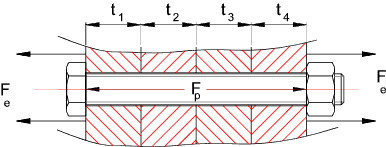Assuming the assembly is bolted with a bolt preload of Fp and an external load F eis applied..
Joint separation will occur when Fe = F (total load on the bolt) .. i.e when no load is being taken by the joint
For an infinitely stiff bolt, separation will never occur as all of the external load will be applied directly to the bolt with no resulting extension..
For a infinitely stiff joint separation will take place when the external load exceeds the preload.

On application of an external force Fe.   Some of the force will used to increase the preload on the bolt and some will be used to reduce the loading on the joint .  The bolt loading diagram below shows the loading regime on the bolt and joint

Bolted Joint diagram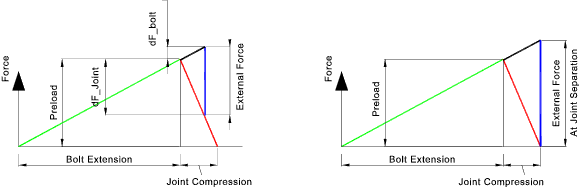The determination of the proportion of the load taken by the bolt and by the joint is calculated using the component stiffness values.
The stiffness is effectively the same as the Spring Rate ..

Stiffness = k = F / δ

Stiffness Considerations

Bolt stiffness

The relationship E = stress /strain = σ /e is used to determine the stiffness of a bolt.
Stress = Force (F) /Area (A) and strain = Deflection (δ) / Length (L)
E = (F/A) /(δ/L) Therefore δ = FL/EA .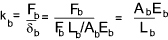If the bolt length clamping the joint includes a number of different sections then the resulting stiffness is determined using the relationship .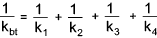To allow for a certain degree of elasticity of the bolt head and nut a correction factor is often used modify the length used in the stiffness calculations as shown below..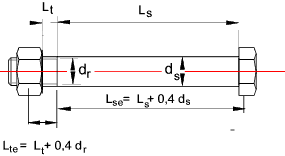The stiffness of the bolt results from the stiffness of the bolt shank (dia ds ) and the stiffness of the bolt thread (root dia dr ).
The length used to calculate the shank stiffness = L se = L s + 0,4d s
The length used for the threaded length section = = L te = L t + 0,4d r

Joint Stiffness

Note: It is very difficult to calculated the stiffness of a joint e.g one based on holes drilled in a plate.  A rough approximation can be made by assuming joint is an annulus with and OD of 2,5 times the bolt dia and an ID = bolt diameter.

Additional notes on evaluating the joint stiffness are provided on page Joint Stiffness

The mitcalc software (links below) is useful for obtaining a stiffness value of a joint.

The relationship E = stress /strain = σ /e is used to determine the stiffness of a section .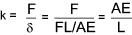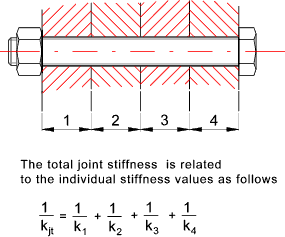Calculation of Load Distribution using Bolt/Joint Stiffness

A joint preloaded with a force Fp is then subject to an additional load Fe which tends to separate the joint.  The resulting deflection of the joint and bolt are the same providing that Fe is less than the separation force.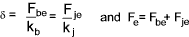It follows that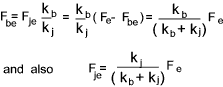Following application of the external force the resulting total force on the bolt =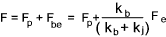and the total force on the joint =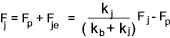If all of the materials of the joint and the bolt are the same then any changes in temperature will have negligible effect of the joint loadings.  However if the joint materials have coefficients of thermal expansion different to the bolt material changes in the joint loading result from changes in temperature...

Let .. Coefficient of thermal expansion of joint material = α j
Coefficient of thermal expansion of bolt = α b
Change of temperature = ΔT = T2- T1

Note: If there is a temperature fall then clearly T1 would be greater that T2 and ΔT would be negative

Length of joint = Length of Bolt ( L j = L b = L )

The expansion of the joint = ΔL j= α j ΔT.L
The expansion of the bolt = ΔL b= α b ΔT.L
The overall stiffness of the joint is calculated as follows.The resulting change in the joint load is calculated as follows..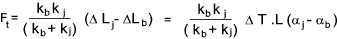The total bolt load following temperature change is thus..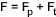Initial Tension in Bolt

The initial tension in a bolt is crudely estimated for a bolt tightened by hand by an experienced mechanic as follows.   The tension resulting from this equation would be reasonable safe for M8- 8,8 grade bolts and above.

Fp = K*d

 d = nominal diameter of bolt (m) Fp = Preload (N) K = Coefficient vary from. 1.75 x 10 6 N/m to 2.8 x 10 6 N /m

For a bolt tightened with a torque wrench the torque required to provide an initial bolt tension may be approximated by the formula..

T = Fp * K * d

Typical K factors

 Steel Thread Condition K as received, stainless on mild or alloy 0,30 as received, mild or alloy on same 0,20 cadmium plated 0,16 molybdenum-disulphide grease 0.14 PTFE lubrication 0.12

A more accurate value can be determined using the formula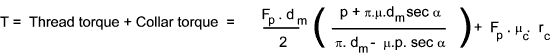Note: Relevant formulea for calculating Power thread torques and efficiencies are derived on webpage Power Screw Equations

 F p = Desired bolt Preload (N) p = Thread pitch (m) d m = Mean diameter of thread (m) μ = Coefficient of Thread friction μ c = Coefficient of collar friction α is the thread angle / 2 (α = 30 o for standard metric threads & α = 29 o/2 for acme threads). r c = Collar friction radius (m)

Note: Friction values are found on this site on the coefficient of friction page..Coefficient of Friction

It can be proved that the majority of the torque is required to overcome the thread and collar friction forces (approx ).   Therefore any error in the value of the friction coefficient will have a large variation on the bolt tensile load.   The above formula is in essence not a lot more accurate than the approximate formulae above.

Note:
A very simplified version of this formula can be derive by assuming μc = μ , d m = 0.92 d , α = 30o. rc = 0.625 d.
If the denominator is simplified to π.dm The equation reduces to

T = Fp (0,159.p + 1,156. μ.d )

This provides a very crude relationship between the torque and the resulting bolt tension for a standard hex screw with no washer..

For important bolting applications it is recommended that the bolt preload is is determined using direct bolt tension measuring techniques - see notes below.

Shear Stress In Bolts

In tightening a bolt stress is induced as a result of the bolt tension and bolt torque..  The combined resulting shear stress is calculated as follows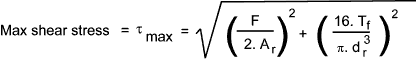F Bolt Tension (N) Tf = Thread torque(N.m) A r = Thread Root Area (m 2) d r = Thread Root diameter

This formula is relatively conservative.  For less conservative designs A r can be replaced by A t as defined on page Thread calcs
and d r can be replaced by d t = Sqrt( 4.A t/π )

In general for static loading, the maximum shear stress in a bolt should not exceed about 75% of the shear yield stress of the material.  For variable loading the bolt should be designed for endurance stress levels.  Bolts subject to dynamic loading often lose their initial torsion stress because the nut/bolt head tends to slip back if the collar friction is not sufficient.

Methods of setting bolt preload /tension

High strength friction grip bolts and nuts require to be tensioned in accordance with BS 4604 which specifies min. loads to be achieved (see Table 1).   It also specifies three methods of determining bolt tension, i.e.:
i) Torque Control
ii) Part Turn of Nut
iii) Direct Tension Measurement

Torque Wrench
This method is sometimes known erroneously as "torque control". The bolts are tensioned in a tension calibrator on the site.   The wrench is then set to cut out at that torque (the wrench becomes "calibrated"), and then all similar bolts that day are installed to that torque after the joint is snugged first.   Rotation during the tightening process must be limited to a specific value.  Actual results of tension in bolts produced by this method are acknowledged to be highly variable, even when this method is followed exactly.

Turn Of Nut
After snugging the joint, the bolt shank and nut is marked and then a specific amount of rotation is induced between the nut and the bolt.   The amount of rotation differs for different bolt lengths and diameters and therefore must be known and understood by the bolt installers in advance.   The success of the method is dependent on a correct snugging of the joint, and is dependent on the bolt head being held from turning so the bolt does not spin in the hole.
Note: Turn-of-nut does not work correctly when the steel surfaces are coated with a compressible coating such as high paint thickness or hot dipped galvanized zinc.

Direct Tension Indicator Washers
A number of direct tension indicator systems are available (see links below) the most popular being the DTI washers. ..The notes below relate to DTI washers..
DTI washers measure the bolt tension developed during tightening, regardless of the torque resistance of the bolt.   By far the simplest method, a DTI washer is put on the head or nut end of the bolt.  The bolts are then slightly tensioned snugging the joint by partially (but not fully) compressing the DTI (tightening the nut).
Then all the bolts are tightened such that the DTI's are "crushed" to the point where a feeler gage cannot be inserted half way around (tightening the nut). DTI's are completely independent of the torque resistance of the bolt assembly.
If the DTI is put on the nut end of the bolt, tightening can be done by one person because it is not necessary access the bolt head side.

Other methods of setting Bolt tension
There are a number of more exotic methods of pre-setting the bolt tension including

 Using Strain gauges..Accurate /complicated /expensive.   Some skill required Using ultrasonics instruments..Accurate and not expensive over the long term.   Some skill required Using special bolts with built-in (mechanical or electronic) extension measurement e.g Rotobolt.    Good but relatively expensive Using hydraulics (or heat) to pretension bolts    See note below Using multi-jackbolt system (Superbolt) a variation of torque control.

Note:
Using heat is based on heating the bolt(and nut) to a set temperature.   The bolt is inserted quickly and the nut tightened snugly.  The bolt is then allowed to cool and the contraction results in the required tension.   If a tension Fp is required for a bolt with a CSA of Ab then the required bolt stress is calculated σ= F p / Ab
The bolt is heated to a temperature of

T = [σ /(E.e)] + T o

E= Youngs Modulus (N/m2 )....e = Coefficient of thermal expansion (m/m)/deg.C....To = ambient temperature (deg. C)

This method is very difficult to implement the bolt has to be heated while the bolted joint has to be kept at ambient temperature. The accuracy of the heating method is very much limited by quality of the procedure followed.

Hydraulic bolt tensioners use an annular hydraulic jack placed around the screw, stretching it axially.   When the required stress level is reached, the nut is tightened snugly and then the pressure released, resulting in a preloaded bolt without any frictional or torsional stresses.

The hydraulic method can provide very accurate preload (+/- 1%) on long bolts but it is less accurate on short bolts.